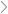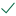Questions & AnswersEconomicsGiven the regression equation...

QuestionAnswered step-by-step

# Given the regression equation...

Given the regression equation

Y=108.811+(−3.968∗First)+(0.732∗Second)+(−8.534∗Third)+(−0.334∗Trend)Y=108.811+(−3.968∗First)+(0.732∗Second)+(−8.534∗Third)+(−0.334∗Trend),

where:

Y=census

First=dummy variable for Q1

Second=dummy variable for Q2

Third=dummy variable for Q3

Trend=variable increasing by 1 unit for each quarter since the data were collected

If we want to forecast (predict) the census for the 20th quarter in the trend, which occurs in the fourth quarter of the year, the value is:

Select one:

a.

102.863

b.

102.131

c.

98.163Solved by verified expert
<p>sectetur adipiscing elit. N</p>sectetur adipiscing elit. Nam lacinia pulvinar tortor nec facilisis. Pellentesque dapibus efficitur laoreet. Nam risus ante, dapibus a molestie consequat, ultrices ac magna. Fusce dui lectus, congue vel laoreet ac, dictum vitae odio. Donec aliquet. Lorem ipsum dolor sit amet, consectetur adipisc10,000 step-by-step explanations